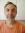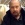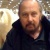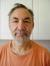# summeringstabell

## English translation: summary table

GLOSSARY ENTRY (DERIVED FROM QUESTION BELOW)
 Swedish term or phrase: summeringstabell English translation: summary table Entered by:

 17:09 Nov 21, 2010
Swedish to English translations [PRO]
Tech/Engineering - Computers: Software / database technology
 Swedish term or phrase: summeringstabell Inget sammanhang, förekommer i lista. Antar att det ska översättas till "aggregation table". Kan någon bekräfta?Local time: 12:11
 summation table Explanation:A mathematical term also used in computing. Please see example below from a Google search. Partial Summation Table The assignment is: ----------------------------------------- This program should print out a table with k rows and m columns. Declare the variables “k” and “m” as constants. Take k=4 and m=5. Each row of the table contains partial sums of jth power of first n integers (1^j+2^j+3^j……..n^j); 1<=n<=m. Proceed this way to the other rows 1<=j<=k. The table should be computing in the following way: 1^1+2^1+...+m^1 | 1^1+2^1+...+(m-1)^1 | 1^1+2^1+...+(m-2)^1 | ...... | 1^1 1^2+2^2+...+m^2 | 1^2+2^2+...+(m-1)^2 | 1^2+2^2+...+(m-2)^2 | ...... | 1^2 1^3+2^3+...+m^3 | 1^3+2^3+...+(m-1)^3 | 1^3+2^3+...+(m-2)^3 | ...... | 1^3 ..... ..... 1^k+2^k+...+m^k | 1^k+2^k+...+(m-1)^k | 1^k+2^k+...+(m-2)^k | ...... | 1^k The Output should look like: 15 10 6 3 1 55 30 14 5 1 225 100 36 9 1 979 354 98 17 1 ----------------------------------------------- My code so far:
Selected response from:

Barry Appleby
United Kingdom
Local time: 12:11
Grading comment
 Thanks also for you message! C2 KudoZ points were awarded for this answer

Summary of answers provided
5summation table
 Barry Appleby4table of sums
 Nils AnderssonDiscussion entries: 2

## Answers

1 hr   confidence:table of sums

Explanation:
Always difficult without context. Other answers are obviously
possible.

 Nils AnderssonUnited StatesWorks in fieldNative speaker of:Swedish,EnglishLogin to enter a peer comment (or grade)

4 hrs   confidence:summation table

Explanation:
A mathematical term also used in computing. Please see example below from a Google search.

Partial Summation Table
The assignment is:
-----------------------------------------
This program should print out a table with k rows and m columns. Declare the variables “k” and “m” as constants. Take k=4 and m=5. Each row of the table contains partial sums of jth power of first n integers (1^j+2^j+3^j……..n^j); 1<=n<=m. Proceed this way to the other rows 1<=j<=k. The table should be computing in the following way:

1^1+2^1+...+m^1 | 1^1+2^1+...+(m-1)^1 | 1^1+2^1+...+(m-2)^1 | ...... | 1^1
1^2+2^2+...+m^2 | 1^2+2^2+...+(m-1)^2 | 1^2+2^2+...+(m-2)^2 | ...... | 1^2
1^3+2^3+...+m^3 | 1^3+2^3+...+(m-1)^3 | 1^3+2^3+...+(m-2)^3 | ...... | 1^3
.....
.....
1^k+2^k+...+m^k | 1^k+2^k+...+(m-1)^k | 1^k+2^k+...+(m-2)^k | ...... | 1^k

The Output should look like:

15 10 6 3 1
55 30 14 5 1
225 100 36 9 1
979 354 98 17 1
-----------------------------------------------

My code so far:

Example sentence(s):
• Summation tables are a mathematical tool that is also used by computer programmers.

Reference: http://www.codeguru.com/forum/showthread.php?t=484240
 Barry ApplebyUnited KingdomLocal time: 12:11Native speaker of: EnglishPRO pts in category: 6Grading comment
 Thanks also for you message! C
Login to enter a peer comment (or grade)

Login or register (free and only takes a few minutes) to participate in this question.

You will also have access to many other tools and opportunities designed for those who have language-related jobs (or are passionate about them). Participation is free and the site has a strict confidentiality policy.

## KudoZ™ translation help

The KudoZ network provides a framework for translators and others to assist each other with translations or explanations of terms and short phrases.

See also:

English

### Select a language

Term search
• All of ProZ.com
• Term search
• Jobs
• Forums
• Multiple search